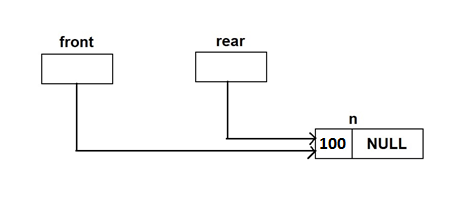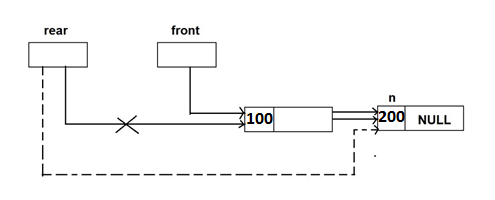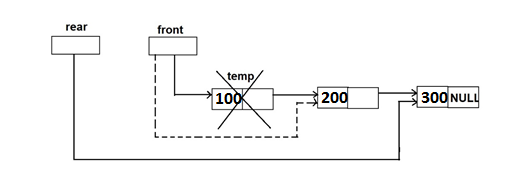A queue can be implemented using linked list. This is known as dynamic implementation of queue.

struct node

{

int info;

struct node *next;

};

typedef struct node NODE;

NODE *front, *rear;

The front and rear will be two pointers pointing to the first and last node respectively. The insert and delete operations on queue are as shown below-

1. Initially

Front=rear=NULL;

1. Insert 100if(front==NULL)

front=rear=n;

1. Insert 200rear->next=n;

rear=n;

1. Delete 100temp=front;

front=front->next;

free(temp);

// Program: Following programs implements queue using linked list

#include<stdio.h>

#include<conio.h>

#include<malloc.h>

struct node

{

int info;

struct node *next;

};

typedef struct node NODE;

NODE *front=null, *rear=NULL;

void main()

{

int ch;

void insert();

void del();

void display();

while(1)

{

clrscr();

printf(“\n 1. Insert”);

printf(“\n 2. Delete”);

printf(“\n 3. Display”);

printf(“\n 4. Quit”);

scanf(“%d”,&ch);

switch(ch)

{

case 1:

insert();

break;

case 2:

del();

break;

case 3:

display();

break;

case 4:

exit(0);

default:

printf(“\n Enter correct choice…”);

}

}

}

void insert()

{

NODE *n;

int data;

n=(NODE*)malloc(sizeof(NODE));

printf(“\n Enter data to insert in queue: ”);

scanf(“%d”,&data);

n->info=data;

n->next=NULL;

if(front==NULL)                                /* if queue is empty */

front=n;

else

rear->next=n;

rear=n;

}

void del()

{

node *temp;

if(front==NULL)

printf(“\n Queue underflow…”);

else

{

temp=front;

printf(“\n Deleted element is %d”,temp->info);

front=front->next;

free(temp);

}

getch();

}

void display()

{

NODE *temp;

temp=front;

if(front==NULL)

printf(“\n Queue is empty…”);

else

{

printf(“\n Queue elements are: ”);

while(temp!=NULL)

{

printf(“%d”,temp->info);

temp=temp->next;

}

printf(“\n”);

}

getch();

}

#### Some More:

POP- Introduction to Programming Using ‘C’

DS – Data structure Using C

OOP – Object Oriented Programming

Java Programming

DBMS – Database Management System

RDBMS – Relational Database Management System